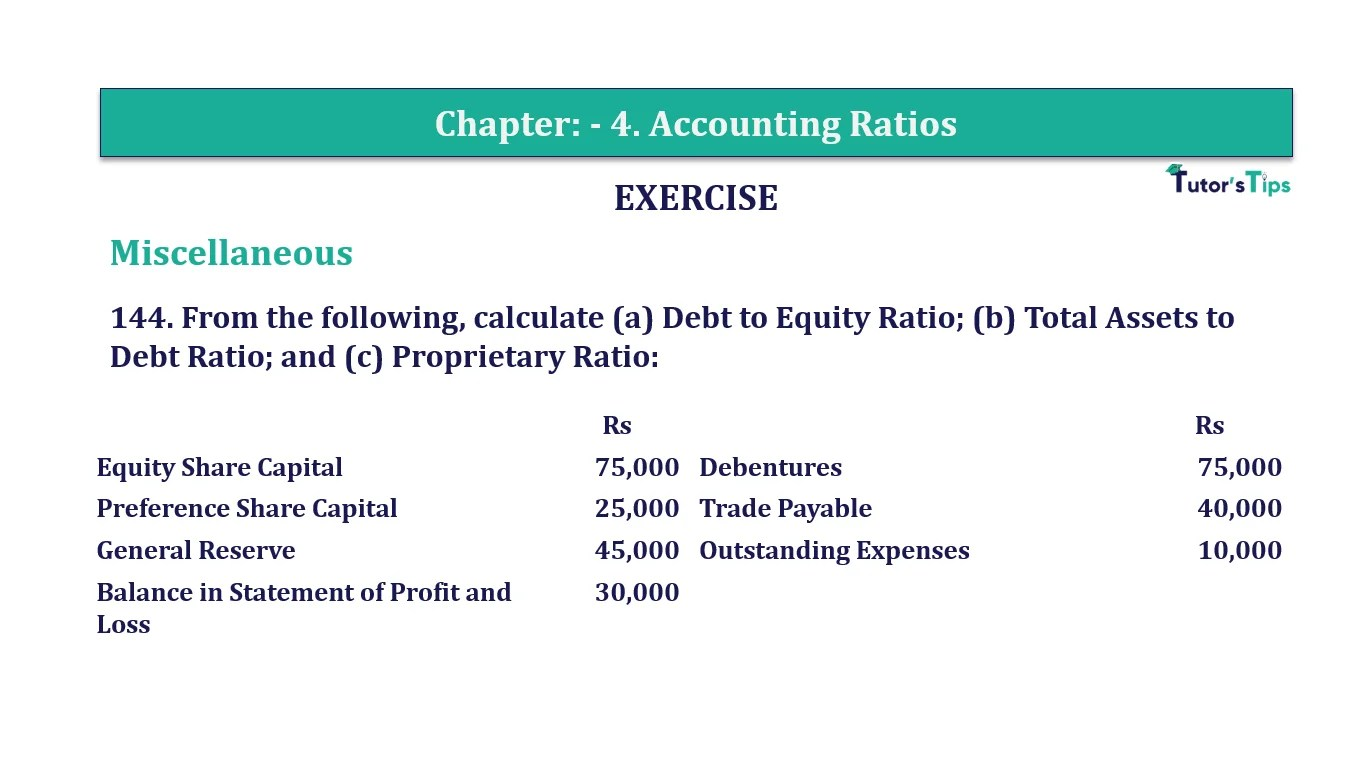# Question 144 Chapter 4 of +2-B – T.S. Grewal 12 ClassQuestion No. 144- Chapter No.4 - T.S. Grewal +2 Book Part B

Question 144 Chapter 4 of +2-B

Miscellaneous

144. From the following, calculate (a) Debt to Equity Ratio; (b) Total Assets to Debt Ratio; and (c) Proprietary Ratio:

 Rs Rs Equity Share Capital 75,000 Debentures 75,000 Preference Share Capital 25,000 Trade Payable 40,000 General Reserve 45,000 Outstanding Expenses 10,000 Balance in Statement of Profit and Loss 30,000

### The solution of Question 144 Chapter 4 of +2-B: –

I

 Long-term Debts = Rs 75,000 Equity = Equity Share Capital + Preference Share Capital + General Reserve + Balance in Statement of Profit & Loss = Rs 75,000 + Rs 25,000 + Rs 45,000 + Rs 30,000 = Rs 1,75,000
 Debt – Equity Ratio = Long-term Debts Equity
 Gross Profit Ratio = Rs. 75,000 Rs 1,75,000 = 0.43 : 1

II

 Total Assets = Equity Share Capital + Preference Share Capital + General Reserve + Balance in Statement of Profit & Loss + Debentures + Trade Payables + Outstanding Expenses = Rs 75,000 + Rs 25,000 +Rs 45,000 + Rs 30,000 + Rs 75,000 + Rs 40,000 + Rs10,000 = Rs 3,00,000 Long term Debts = Rs 75,000
 Total Assets to Debts Ratio = Total Assets Long term Debts
 = Rs. 3,00,000 Rs 75,000 = 4 : 1

III

 Shareholders’ Funds = Equity Share Capital + Preference Share Capital + General Reserve + Balance in Statement of Profit & Loss = 75,000+25,000+45,000+30,000 = Rs 1,75,000 Total Assets = Equity Share Capital + Preference Share Capital + General Reserve +Balance in Statement of Profit & Loss + Debentures + Trade Payables + Outstanding Expenses = Rs 75,000 + Rs 25,000 + Rs 45,000 + Rs 30,000 + Rs 75,000 + Rs 40,000 + Rs 10,000 = Rs 3,00,000
 Proprietary Ratio = Shareholders’ Funds Total Assets
 = Rs. 1,75,000 Rs 3,00,000 = 0.58 : 1

Comment if you have any question.

Also, Check out the solved question of previous Chapters: –